# ISEE Middle Level Quantitative : How to subtract fractions

## Example Questions

1 2 3 4 5 6 8 Next →

### Example Question #35 : How To Subtract Fractions

Zach cleanedof the house and Alex cleanedof the house. How much more of the house did Alex clean?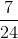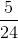Explanation: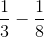In order to solve this problem, we first need to make common denominators.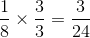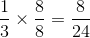Now that we have common denominators, we can subtract the fractions. Remember, when we subtract fractions, the denominator stays the same, we only subtract the numerator.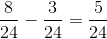### Example Question #36 : How To Subtract Fractions

Lily pulledof the weeds and Rose pulled. How much more of the weeds did Rose pull?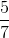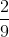Explanation: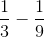In order to solve this problem, we first need to make common denominators.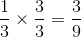Now that we have common denominators, we can subtract the fractions. Remember, when we subtract fractions, the denominator stays the same, we only subtract the numerator.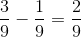### Example Question #37 : How To Subtract Fractions

Sally drank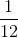of the milk and Sam drank. How much more of the milk did Sam drink?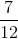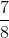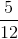Explanation: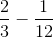In order to solve this problem, we first need to make common denominators.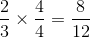Now that we have common denominators, we can subtract the fractions. Remember, when we subtract fractions, the denominator stays the same, we only subtract the numerator.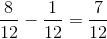### Example Question #38 : How To Subtract Fractions

Jake ate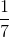of the popcorn and Dave ate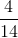of the popcorn. How much more of the popcorn did Dave eat?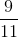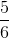Explanation: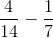In order to solve this problem, we first need to make common denominators.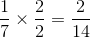Now that we have common denominators, we can add the fractions. Remember, when we add fractions, the denominator stays the same, we only add the numerator.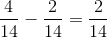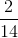can be reduced by dividingby both sides.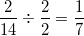1 2 3 4 5 6 8 Next →